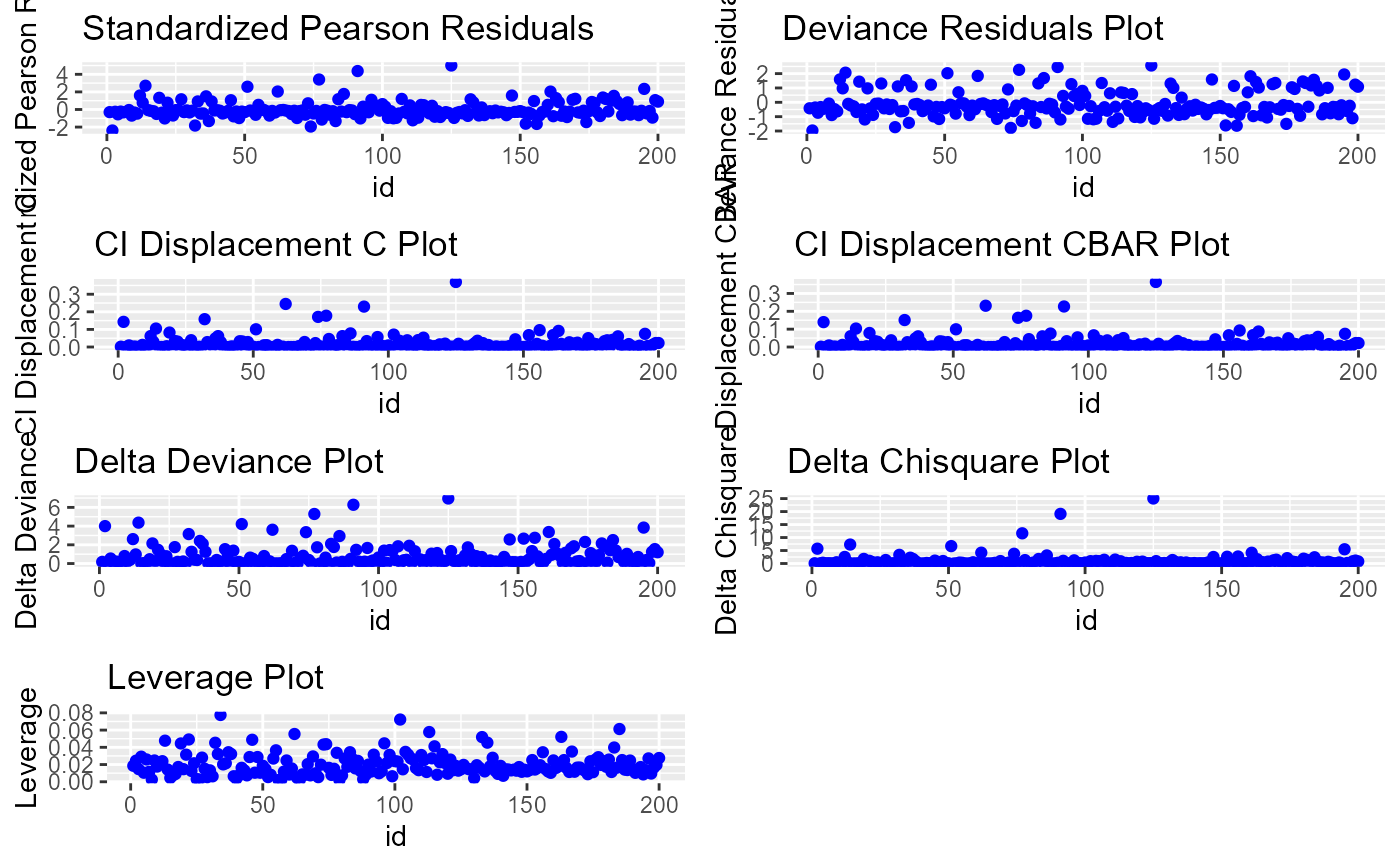Reisudal diagnostic plots for detecting influential observations.

blr_plot_diag_influence(model, print_plot = TRUE)

## Arguments

model An object of class glm. logical; if TRUE, prints the plot else returns a plot object.

## Value

A panel of influence diagnostic plots.

Fox, John (1991), Regression Diagnostics. Newbury Park, CA: Sage Publications.

Cook, R. D. and Weisberg, S. (1982), Residuals and Influence in Regression, New York: Chapman & Hall.

Other diagnostic plots: blr_plot_diag_fit(), blr_plot_diag_leverage()
model <- glm(honcomp ~ female + read + science, data = hsb2,